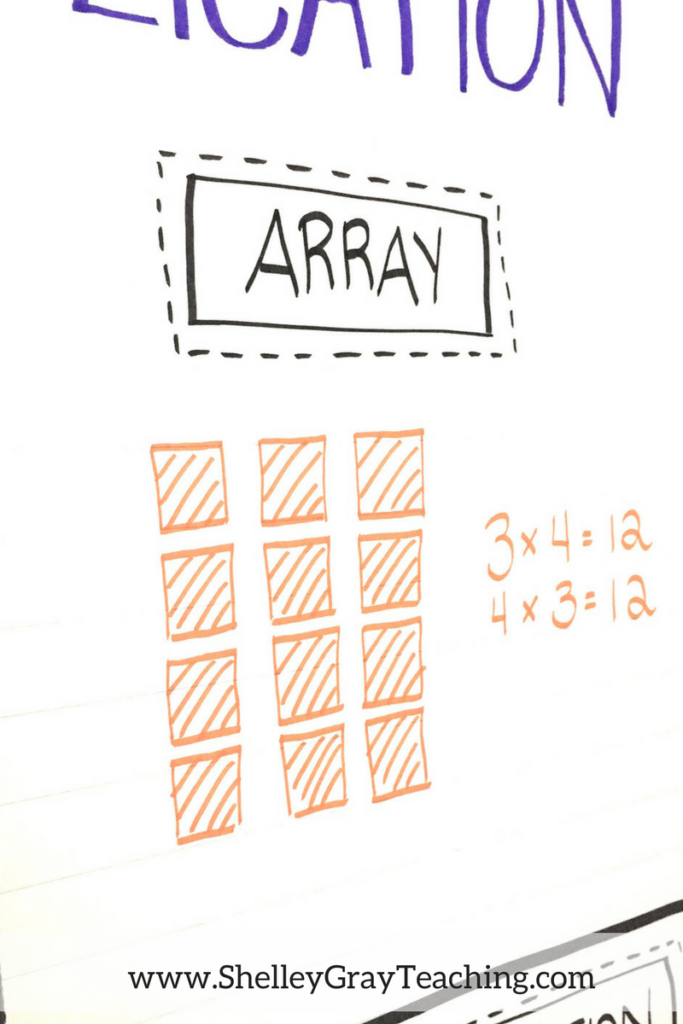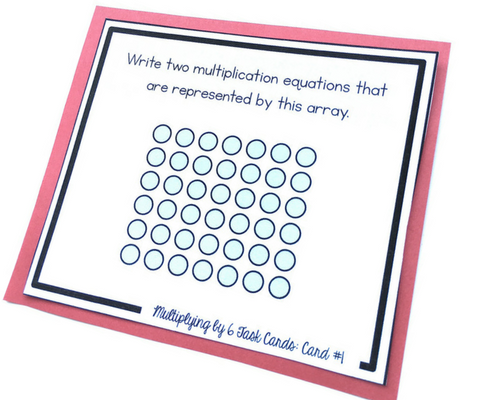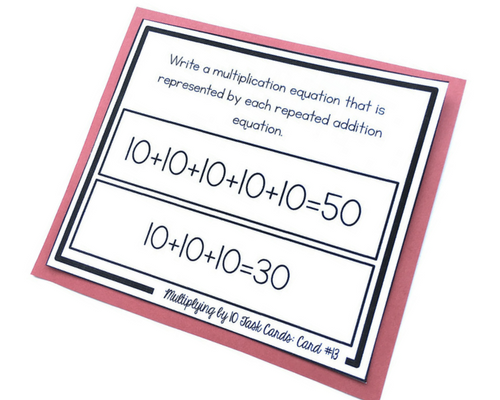# Four Ways to Represent MultiplicationWhen it comes to teaching multiplication, it can be tricky to know how to approach it in your classroom. Often we teach multiplication using the “equal groups” idea and leave it at that. But it is important to teach multiplication using different approaches and strategies so students understand it in many different ways.

Here are four ways to teach multiplication in your classroom. Make sure that these are all included in your instruction so that your students are able to visualize multiplication in different ways while they learn what it really means. Once students REALLY understand multiplication, you’ll be able to move away from these approaches and focus more on multiplication fact mastery.

## REPRESENT MULTIPLICATION USING ARRAYSAn array is a group of objects that is arranged into rows and columns. This group of objects can be used to represent a multiplication equation by multiplying the number of rows by the number of columns. For example, the array below shows 6 rows and 7 columns. This represents the multiplication equation 6×7 or 7×6. Altogether there are 42 objects in this array.###### FIND THIS TASK CARD IN THE BASIC MULTIPLICATION TASK CARD BUNDLE HERE.

Ask students to identify the equation represented by an array, as well as draw arrays to represent multiplication equations.

## SKIP COUNTING TO REPRESENT MULTIPLICATIONSkip-counting is generally an introductory activity for multiplication. Students can use a number line, or a simple skip-counting sequence to figure out the product of an equation. For example, to figure out the product of 5 and 4, we can skip-count 4 groups of 5 – 5, 10, 15, 20 or 5 groups of 4 – 4, 8, 12, 16, 20.

## FREE MULTIPLICATION ACTIVITIESWhen we teach multiplication, we want our students to understand that it can be used as a shortcut to addition. For example, to figure out the problem below, we could add 8+8+8+8+8 to make 40 and get the correct answer for this problem. However, it is much faster to multiply 5×8 to make 40 instead.###### FIND THIS TASK CARD IN THE BASIC MULTIPLICATION TASK CARD BUNDLE HERE.

We can ask our students to look at a repeated addition equation and identify the multiplication equation that is represented.## PICTURE REPRESENTATIONS/EQUAL GROUPSEqual groups are a great way to introduce multiplication. The idea of equal groups can be understood more when introduced with a picture. This task card shows the equation 6×8 represented in an “equal groups” picture. Be sure to have your students draw picture representations that make sense to them, as well as identify the equation represented by a picture.## NEXT STEPS

• Create a multiplication anchor chart like the one shown here to post in your classroom:•Thank you Shelly! That is very helpful. I used it and I am sharing it with a friend.

••